1 Tutorials that teach Working with Data from Multiple Samples

# Working with Data from Multiple Samples

Author: Sophia Tutorial
##### Description:

Understand the relationship between the mean of sample means and the population mean.

(more)

Sophia’s self-paced online courses are a great way to save time and money as you earn credits eligible for transfer to many different colleges and universities.*

No credit card required

46 Sophia partners guarantee credit transfer.

299 Institutions have accepted or given pre-approval for credit transfer.

* The American Council on Education's College Credit Recommendation Service (ACE Credit®) has evaluated and recommended college credit for 33 of Sophia’s online courses. Many different colleges and universities consider ACE CREDIT recommendations in determining the applicability to their course and degree programs.

Tutorial
what's covered
This lesson is on working with data from multiple samples. By the end of this lesson, you should be able to understand that sample means better approximate population means as the sample sizes get larger. This lesson covers:
1. Random Sampling
2. Distribution of Sample Means

## 1. Random Sampling

Recall that a sample is a selection of observations from a larger group, which is known as a population. The idea behind taking a sample is that it is substantially easier to obtain a small number of observations than it is to obtain all observations. The sample should be randomly drawn so as to improve the probability of the sample observations being representative of the population. This is due to the results of random sample being subject to chance. It does not provide the observer with an exact estimate of what the population looks like.

Also recall that you use the mean or average of a group of observations to determine the center of a data set. Regardless of whether this data is a sample or a population, the mean is still a useful measure of center. However, you cannot expect the mean of a random sample and the mean of the population to be the same, since a sample is only a portion of the population.

IN CONTEXT

Suppose that you were to select 100 random people on a college campus and ask them their age. Is it possible that the mean age of our sample would equal the mean age of everyone on campus? Probably not. Why is this?

Maybe many of the 100 people you asked happen to randomly be freshman. The mean is likely to be less than that of the overall population of the college. Maybe you randomly sampled a lot of faculty members, so the mean of the sample was actually higher than that of the campus population.

## 2. Distribution of Sample Means

Typically, the mean of one sample is not itself an accurate representative of the entire population. Because of this, you need to take multiple samples and then find the mean of all the samples that you take. This approach generally provides more accurate data than simply taking one sample.

When you collect a lot of random samples you will get a lot of sample means, one for each sample. Keep in mind that there is likely to be a difference between how the sample means that you collect are distributed relative to the population mean. It is still possible that even if you collect multiple samples that none of the sample means will be close to the population mean. Generally, by collecting more samples you will get a mean for each sample. Looking at the mean of all the sample makes the estimates of the population tend to be more accurate.

It is important to realize that the shape of the distribution of the sample means is normally distributed, meaning that they follow a bell-shaped curve of which the center is the population mean. If the samples we randomly draw are large enough, the distribution of the sample means will approach a normal distribution that is centered on the population mean.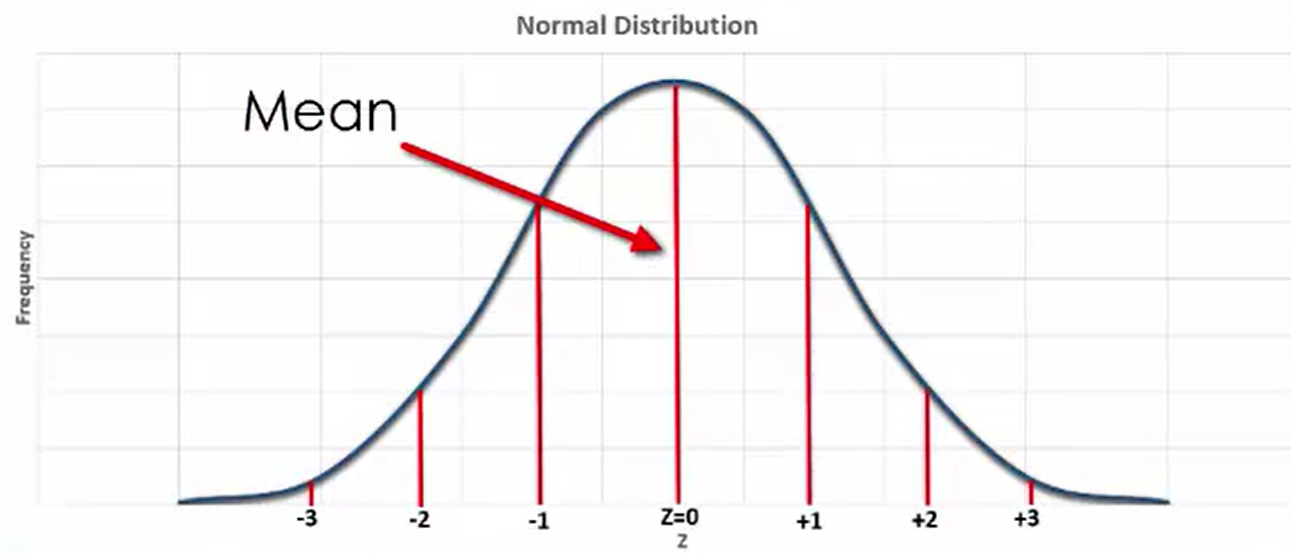Remember that the standard deviation is a measurement of how much a data set varies from the mean, or how spread out the data is. A relatively high standard deviation indicates that the data is more spread out than if the standard deviation were lower.

In the event that the sample sizes are small, the sample means will typically be more spread out from the center of the distribution, or possibly not even normally distributed at all.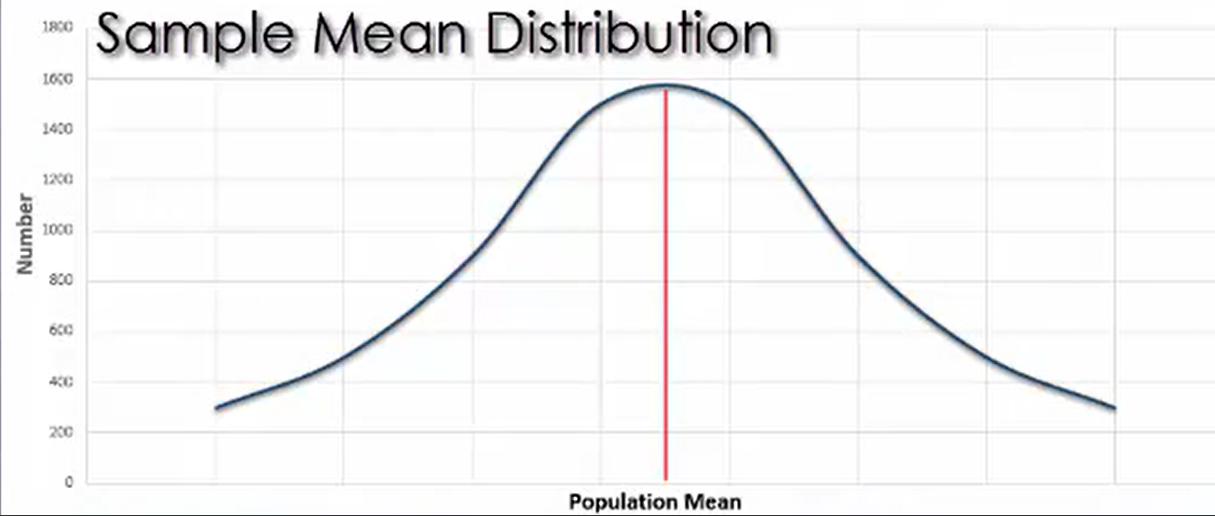If the sample sizes are large, on the other hand, the sample means will tend to be clustered close to the center of the distribution. When the sample distribution needs to be close to a normal distribution, you need to draw more samples.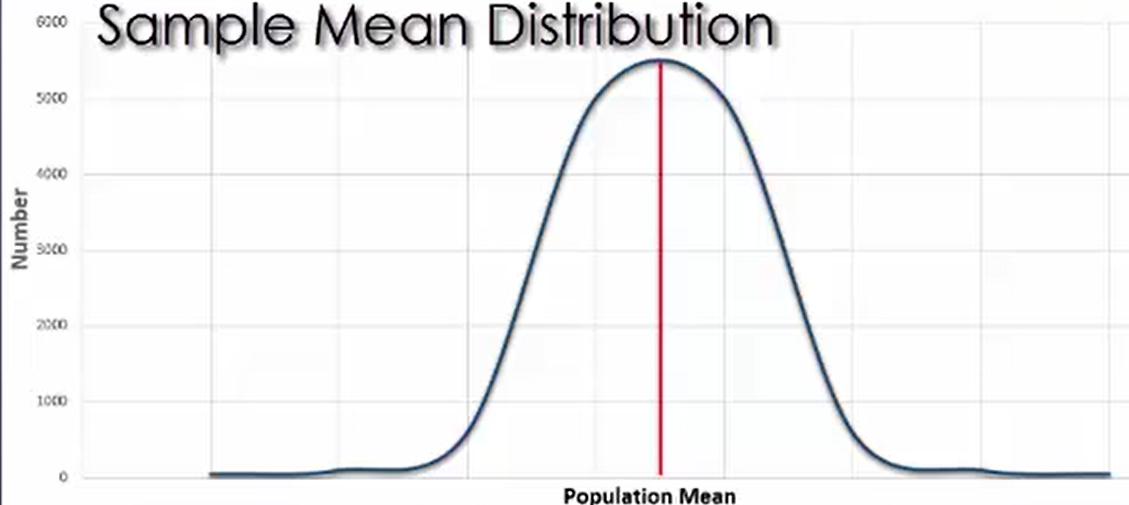Just like you can determine a z-score for individual values, you can also determine the z-score of a mean. By using a z-distribution graph, the means whose z-scores are closer to zero are more likely to occur in a sample.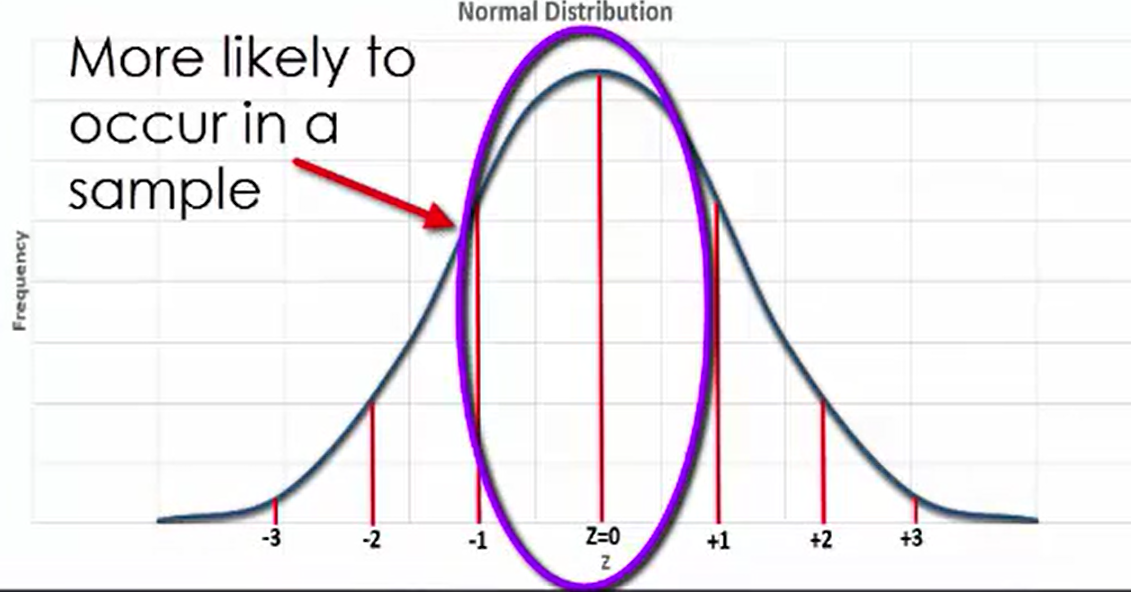The means that have z-scores farther from zero are less likely to occur in a sample.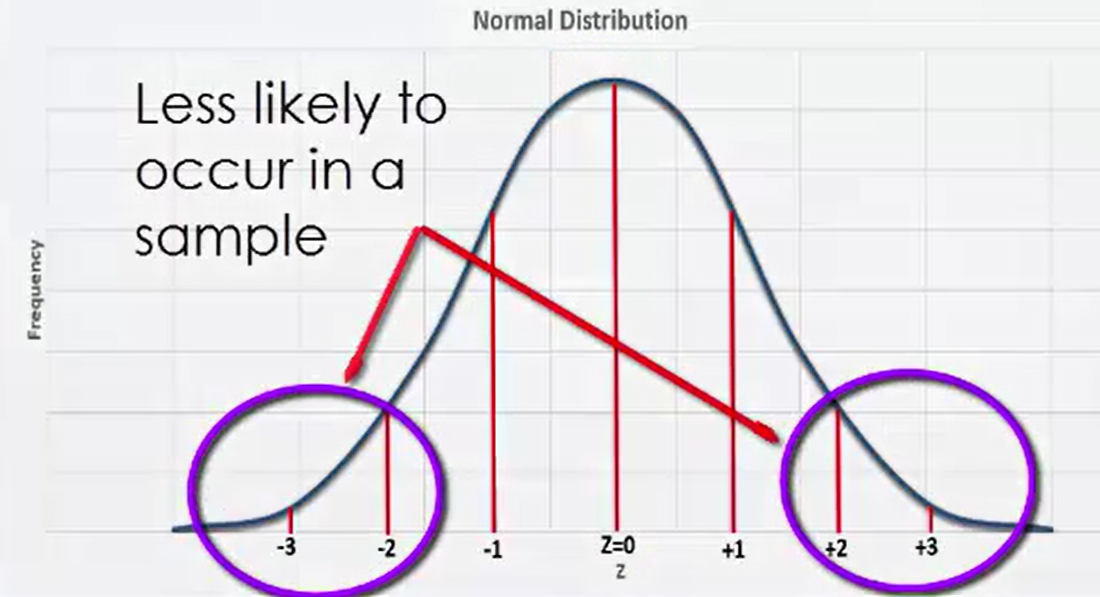IN CONTEXT

Suppose that you know the mean number of children per classroom in American elementary schools is 27, with a standard deviation of 3. Additionally, assume that the population distribution in this scenario is not normally distributed. As you can see in this graph, a sample of 100 schools indicates that the standard deviation of the sample is 5.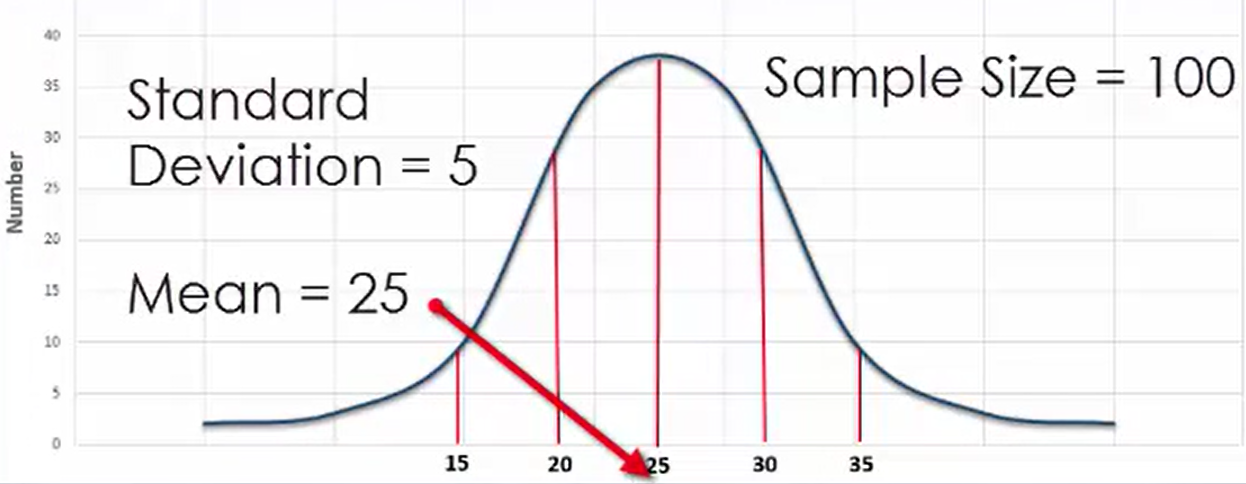As you continue to look at more samples, you can see that as the sample size changes, the shape of the sample distribution changes as well: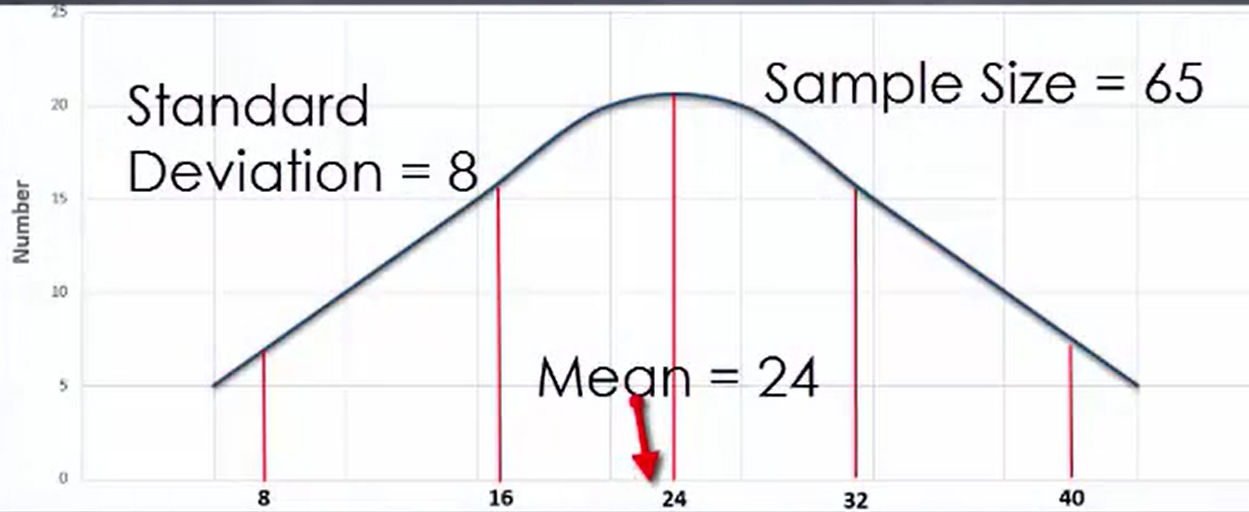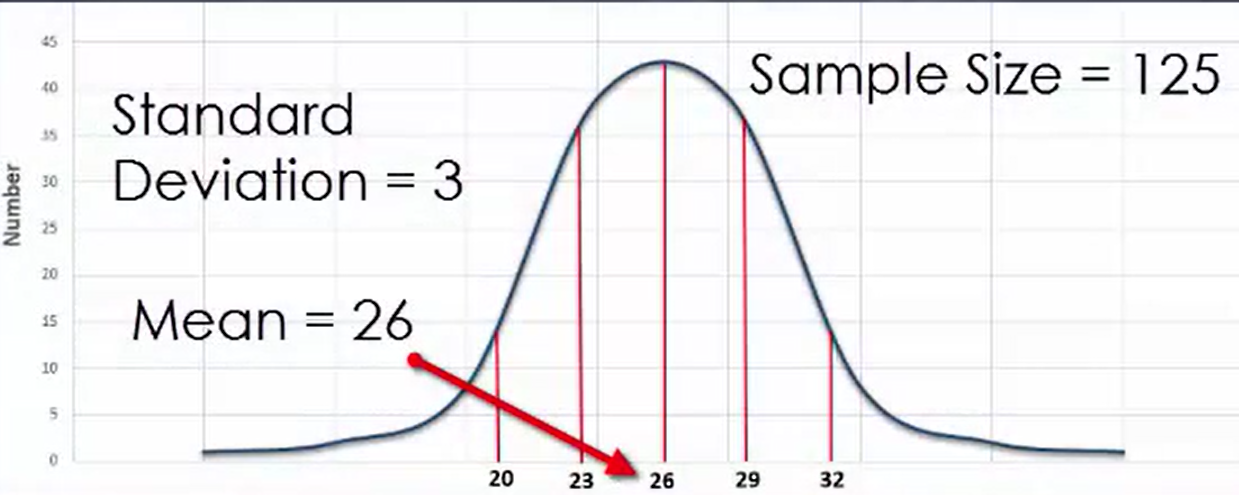Notice in particular that smaller samples result in a wider distribution, while larger samples result in a narrower distribution. The sample means of four of the samples are 25.3, 28.9, 27.9, and 26.8 students per class.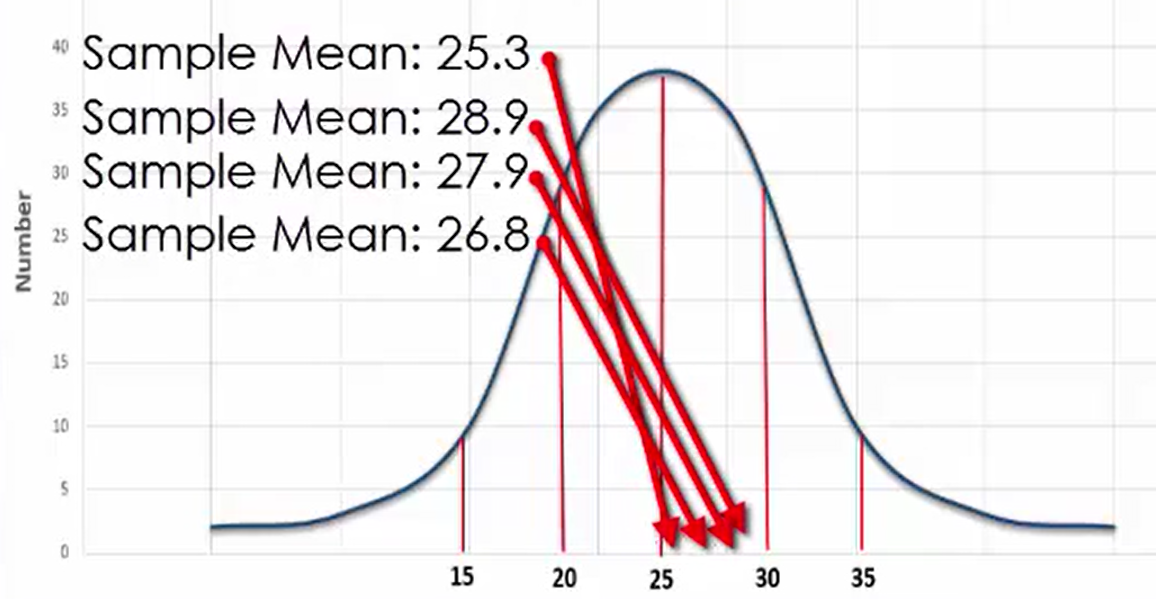summary
It is important to conduct random sampling to get a more accurate representation of the population as a whole. However, since you are still working with a sample, it still may not match the population. It is important to understand that sample means better approximate population means as the sample sizes get larger. Distribution of sample means reflect a normal distribution the larger the sample size is.

Source: This work is adapted from Sophia author Dan Laub

Rating